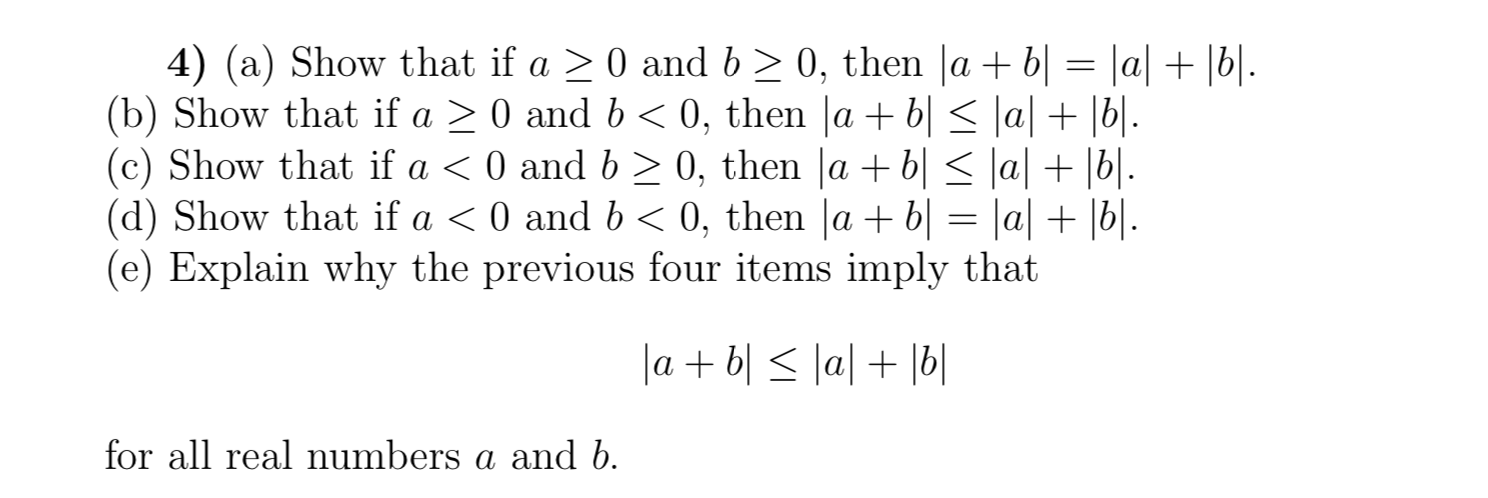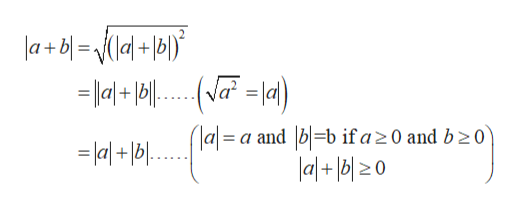# 4) (a) Show that if a 0 and b 2 0, then |a + b| = |a| + |b|.(b) Show that if a(c) Show that if a < 0 and b 2 0, then |a b| < \a| + |b|.(d) Show that if a < 0 and b(e) Explain why the previous four items imply that0, then |a + b| < \a| + |b|..0 and b0, then lab= |a| + |b|.labllal beal numbers a and bfor all

Question
21 views

Hello, I am confused on how to prove this question.help_outlineImage Transcriptionclose4) (a) Show that if a 0 and b 2 0, then |a + b| = |a| + |b|. (b) Show that if a (c) Show that if a < 0 and b 2 0, then |a b| < \a| + |b|. (d) Show that if a < 0 and b (e) Explain why the previous four items imply that 0, then |a + b| < \a| + |b|.. 0 and b 0, then lab= |a| + |b|. labllal b eal numbers a and b for all fullscreen
check_circle

Step 1

(a)By definition, we have

Step 2

Now,

Step 3

After solvi...help_outlineImage Transcriptionclosea+bl=\{Ia|+l6|) * (la= a and b=b if a 20 and b 2 0 lal+bl0 = la+bl fullscreen

### Want to see the full answer?

See Solution

#### Want to see this answer and more?

Solutions are written by subject experts who are available 24/7. Questions are typically answered within 1 hour.*

See Solution
*Response times may vary by subject and question.
Tagged in

### Algebra